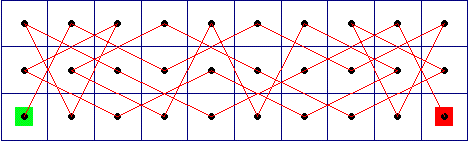## The Distribution of the Knight

Dr Colin Rose

The Knight's Tour problem can be stated as follows:

• Given a chess board with n × m squares, find a path for the knight that visits every square exactly once.

For example, here is a solution to the knight's tour problem on a 3 × 10 chess board. In this example, the knight starts out in the lower left corner and ends in the bottom right corner:If we started on the second square on the first row, would it still be possible to find a solution? More generally, what does the distribution of solutions across the chess board look like? That is, for every given starting point, how many solutions exist?

This problem can be solved by computer: for every given starting point, evaluate every possible path that visits each square exactly once, and then count how many solutions exist. Mathematica was enlisted to help with the hunt ...

We present here the distribution of solutions across a chess board, for all chess boards with 32 or less squares.

### Select a chess board from the menu on the left.

Related Links